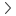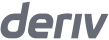# Stop loss and/or take profit calculator for margin

Traders' toolsPnL for margin

Sell

0
0
0
0

Synthetic

Financial

0
0
0
0

Synthetic

Financial

### How to calculate stop loss and/or take profit level and pip value

The stop loss and/or take profit level and pip value when buying a contract on Deriv MT5 is calculated based on the formula:

Stop loss and/or take profit level = asset price + {stop loss OR take profit amount ÷ (volume × contract size)}

Stop loss and/or take profit pip value = |(stop loss OR take profit level - asset price)| ÷ point value

The stop loss and/or take profit level helps you to manage your risk when you are buying a contract.

For financial accounts, the stop loss and/or take profit pip value is in the quote currency for forex pairs.

For synthetic accounts, the stop loss and/or take profit pip value is in USD.

### Example calculation

Let’s say you want to calculate the stop loss level and pip value when you want to buy a lot of EUR/USD priced at 1.17524 USD with a stop loss amount of 24 USD.

1.17524
Asset price
+
{ -24
Stop loss or take profit amount
÷
( 1
Volume
x
100,000 ) }
Contract size 1
=
1.1750
Stop loss level
1.17524
Asset price
+
{ -24
profit amount
Stop loss or take
÷
( 1
Volume
x
100,0001 ) }
Contract size 1
=
1.1750
Stop loss level
1.17524
Asset price
+
{ -24
Stop loss or take profit amount
÷
( 1
Volume
x
100,000 ) }
Contract size 1
=
1.1750
Stop loss level
1.17524
Asset price
+
{ -24
profit amount
Stop loss or take
÷
( 1
Volume
x
100,0001 ) }
Contract size 1
=
1.1750
Stop loss level
1. The contract size is one standard lot of forex = 100,000 units.
|(1.1750
Stop loss or take profit level
-
1.17524)|
Asset price
÷
0.00001
Point value 1
=
24
Stop loss pip value
|( 1.1750
take profit level
Stop loss or
-
1.17524)|
Asset Price
÷
0.00001
Point value 1
=
24
Stop loss pip value
|(1.1750
Stop loss or take profit level
-
1.17524)|
Asset price
÷
0.00001
Point value 1
=
24
Stop loss pip value
|( 1.1750
take profit level
Stop loss or
-
1.17524)|
Asset Price
÷
0.00001
Point value 1
=
24
Stop loss pip value
1. The point value is derived from the current digits of the asset. In this example, the digit is 5, so the point value is 0.00001.

Your position is automatically closed when your stop loss level is at 1.1750 and when your stop loss pip value is 24 USD.